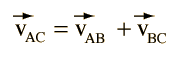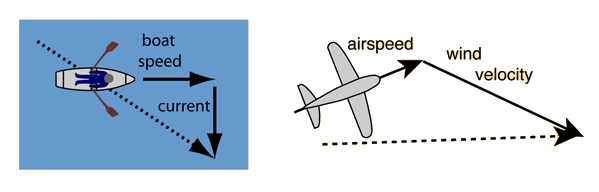# Relative Motion

The laws of physics which apply when you are at rest on the earth also apply when you are in any reference frame which is moving at a constant velocity with respect to the earth. For example, you can toss and catch a ball in a moving bus if the motion is in a straight line at constant speed.

The motion may have a different appearance as viewed from a different reference frame, but this can be explained by including the relative velocity of the reference frame in the description of the motion.

Index

 HyperPhysics***** Mechanics R Nave
Go Back

# Relative Velocity

One must take into account relative velocities to describe the motion of an airplane in the wind or a boat in a current. Assessing velocities involves vector addition and a useful approach to such relative velocity problems is to think of one reference frame as an "intermediate" reference frame in the form:Put into words, the velocity of A with respect to C is equal to the velocity of A with respect to B plus the velocity of B with respect to C. Reference frame B is the intermediate reference frame. This approach can be used with the airplane or boat examples.Index

 HyperPhysics***** Mechanics R Nave
Go Back